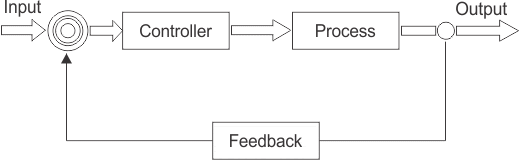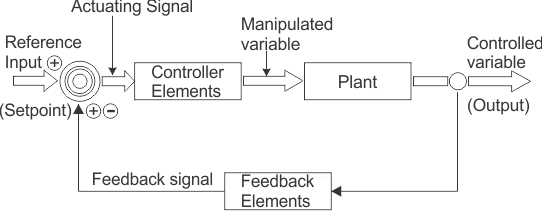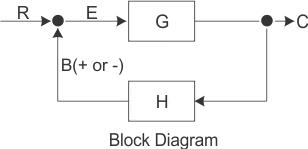Closed-loop Control System This sensor would monitor the actual dryness of the clothes and compare it with (or subtract it...

### Closed-loop Control SystemThis sensor would monitor the actual dryness of the clothes and compare it with (or subtract it from) the input reference. The error signal (error = required dryness – actual dryness) is amplified by the controller, and the controller output makes the necessary correction to the heating system to reduce any error. For example if the clothes are too wet the controller may increase the temperature or drying time. Likewise, if the clothes are nearly dry it may reduce the temperature or stop the process so as not to overheat or burn the clothes, etc.
Then the closed-loop configuration is characterised by the feedback signal, derived from the sensor in our clothes drying system. The magnitude and polarity of the resulting error signal, would be directly related to the difference between the required dryness and actual dryness of the clothes.
Also, because a closed-loop system has some knowledge of the output condition, (via the sensor) it is better equipped to handle any system disturbances or changes in the conditions which may reduce its ability to complete the desired task.
For example, as before, the dryer door opens and heat is lost. This time the deviation in temperature is detected by the feedback sensor and the controller self-corrects the error to maintain a constant temperature within the limits of the preset value. Or possibly stops the process and activates an alarm to inform the operator.
As we can see, in a closed-loop control system the error signal, which is the difference between the input signal and the feedback signal (which may be the output signal itself or a function of the output signal), is fed to the controller so as to reduce the systems error and bring the output of the system back to a desired value. In our case the dryness of the clothes. Clearly, when the error is zero the clothes are dry.
The term Closed-loop control always implies the use of a feedback control action in order to reduce any errors within the system, and its “feedback” which distinguishes the main differences between an open-loop and a closed-loop system.The accuracy of the output thus depends on the feedback path, which in general can be made very accurate and within electronic control systems and circuits, feedback control is more commonly used than open-loop or feed forward control.
Closed-loop systems have many advantages over open-loop systems. The primary advantage of a closed-loop feedback control system is its ability to reduce a system’s sensitivity to external disturbances, for example opening of the dryer door, giving the system a more robust control as any changes in the feedback signal will result in compensation by the controller.
Then we can define the main characteristics of Closed-loop Control as being:
• To reduce errors by automatically adjusting the systems input.
• To improve stability of an unstable system.
• To increase or reduce the systems sensitivity.
• To enhance robustness against external disturbances to the process.
• To produce a reliable and repeatable performance.
Whilst a good closed-loop system can have many advantages over an open-loop control system, its main disadvantage is that in order to provide the required amount of control, a closed-loop system must be more complex by having one or more feedback paths. Also, if the gain of the controller is too sensitive to changes in its input commands or signals it can become unstable and start to oscillate as the controller tries to over-correct itself, and eventually something would break. So we need to “tell” the system how we want it to behave within some pre-defined limits.

## Closed-loop Summing Points

For a closed-loop feedback system to regulate any control signal, it must first determine the error between the actual output and the desired output. This is achieved using a summing point, also referred to as a comparison element, between the feedback loop and the systems input. These summing points compare a systems set point to the actual value and produce a positive or negative error signal which the controller responds too. where: Error = Set point – ActualThe symbol used to represent a summing point in closed-loop systems block-diagram is that of a circle with two crossed lines as shown. The summing point can either add signals together in which a Plus ( + ) symbol is used showing the device to be a “summer” (used for positive feedback), or it can subtract signals from each other in which case a Minus (  ) symbol is used showing that the device is a “comparator” (used for negative feedback) as shown.

### Summing Point TypesNote that summing points can have more than one signal as inputs either adding or subtracting but only one output which is the algebraic sum of the inputs. Also the arrows indicate the direction of the signals. Summing points can be cascaded together to allow for more input variables to be summed at a given point.

## Closed-loop System Transfer Function

The Transfer Function of any electrical or electronic control system is the mathematical relationship between the systems input and its output, and hence describes the behaviour of the system. Note also that the ratio of the output of a particular device to its input represents its gain. Then we can correctly say that the output is always the transfer function of the system times the input. Consider the closed-loop system below.

### Typical Closed-loop System RepresentationWhere: block G represents the open-loop gains of the controller or system and is the forward path, and block H represents the gain of the sensor, transducer or measurement system in the feedback path.
To find the transfer function of the closed-loop system above, we must first calculate the output signal θo in terms of the input signal θi. To do so, we can easily write the equations of the given block-diagram as follows.
The output from the system is equal to:    Output = G x Error
Note that the error signal, θe is also the input to the feed-forward block:  G
The output from the summing point is equal to:    Error = Input - H x Output
If  H = 1 (unity feedback) then:
The output from the summing point will be:    Error (θe) = Input - Output
Eliminating the error term, then:
The output is equal to:    Output = G x (Input - H x Output)
Therefore:    G x Input = Output + G x H x Output
Rearranging the above gives us the closed-loop transfer function of:The above equation for the transfer function of a closed-loop system shows a Plus ( + ) sign in the denominator representing negative feedback. With a positive feedback system, the denominator will have a Minus (  ) sign and the equation becomes:  1 - GH.
We can see that when  H = 1 (unity feedback) and G is very large, the transfer function approaches unity as:Also, as the systems steady state gain G decreases, the expression of:  G/(1 + G)decreases much more slowly. In other words, the system is fairly insensitive to variations in the systems gain represented by G, and which is one of the main advantages of a closed-loop system.

## Multi-loop Closed-loop System

Whilst our example above is of a single input, single output closed-loop system, the basic transfer function still applies to more complex multi-loop systems. Most practical feedback circuits have some form of multiple loop control, and for a multi-loop configuration the transfer function between a controlled and a manipulated variable depends on whether the other feedback control loops are open or closed.
Consider the multi-loop system below.Any cascaded blocks such as G1 and G2 can be reduced, as well as the transfer function of the inner loop as shown.After further reduction of the blocks we end up with a final block diagram which resembles that of the previous single-loop closed-loop system.And the transfer function of this multi-loop system becomes:Then we can see that even complex multi-block or multi-loop block diagrams can be reduced to give one single block diagram with one common system transfer function.

## Closed-loop Motor Control

So how can we use Closed-loop Systems in Electronics. Well consider our DC motor controller from the previous open-loop tutorial. If we connected a speed measuring transducer, such as a tachometer to the shaft of the DC motor, we could detect its speed and send a signal proportional to the motor speed back to the amplifier. A tachometer, also known as a tacho-generator is simply a permanent-magnet DC generator which gives a DC output voltage proportional to the speed of the motor.
Then the position of the potentiometers slider represents the input, θi which is amplified by the amplifier (controller) to drive the DC motor at a set speed Nrepresenting the output, θo of the system, and the tachometer T would be the closed-loop back to the controller. The difference between the input voltage setting and the feedback voltage level gives the error signal as shown.

### Closed-loop Motor ControlAny external disturbances to the closed-loop motor control system such as the motors load increasing would create a difference in the actual motor speed and the potentiometer input set point.
This difference would produce an error signal which the controller would automatically respond too adjusting the motors speed. Then the controller works to minimize the error signal, with zero error indicating actual speed which equals set point.
Electronically, we could implement such a simple closed-loop tachometer-feedback motor control circuit using an operational amplifier (op-amp) for the controller as shown.

### Closed-loop Motor Controller CircuitThis simple closed-loop motor controller can be represented as a block diagram as shown.

### Block Diagram for the Feedback ControllerA closed-loop motor controller is a common means of maintaining a desired motor speed under varying load conditions by changing the average voltage applied to the input from the controller. The tachometer could be replaced by an optical encoder or Hall-effect type positional or rotary sensor.

## Closed-loop Systems Summary

We have seen that an electronic control system with one or more feedback paths is called a Closed-loop System. Closed-loop control systems are also called “feedback control systems” are very common in process control and electronic control systems. Feedback systems have part of their output signal “fed back” to the input for comparison with the desired set point condition. The type of feedback signal can result either in positive feedback or negative feedback.
In a closed-loop system, a controller is used to compare the output of a system with the required condition and convert the error into a control action designed to reduce the error and bring the output of the system back to the desired response. Then closed-loop control systems use feedback to determine the actual input to the system and can have more than one feedback loop.
Closed-loop control systems have many advantages over open-loop systems. One advantage is the fact that the use of feedback makes the system response relatively insensitive to external disturbances and internal variations in system parameters such as temperature. It is thus possible to use relatively inaccurate and inexpensive components to obtain the accurate control of a given process or plant.
However, system stability can be a major problem especially in badly designed closed-loop systems as they may try to over-correct any errors which could cause the system to loss control and oscillate.

#### Control system in which the output has an effect on the input quantity in such a manner that the input quantity will adjust itself based on the output generated is called closed loop control system. Open loop control system can be converted in to closed loop control system by providing a feedback. This feedback automatically makes the suitable changes in the output due to external disturbance. In this way closed loop control system is called automatic control system. Figure below shows the block diagram of closed loop control system in which feedback is taken from output and fed in to input.Practical Examples of Closed Loop Control System
1.Automatic Electric Iron – Heating elements are controlled by output temperature of the iron.
2.Servo Voltage Stabilizer – Voltage controller operates depending upon output voltage of the system.
3.Water Level Controller– Input water is controlled by water level of the reservoir.
4.Missile Launched & Auto Tracked by Radar – The direction of missile is controlled by comparing the target and position of the missile.
5.An Air Conditioner – An air conditioner functions depending upon the temperature of the room.
6.Cooling System in Car – It operates depending upon the temperature which it controls.
Advantages of Closed Loop Control System
1.Closed loop control systems are more accurate even in the presence of non-linearity.
2.Highly accurate as any error arising is corrected due to presence of feedback signal.
3.Bandwidth range is large.
4.Facilitates automation.
5.The sensitivity of system may be made small to make system more stable.
6.This system is less affected by noise.
Disadvantages of Closed Loop Control System
1.They are costlier.
2.They are complicated to design.
3.Required more maintenance.
5.Overall gain is reduced due to presence of feedback.
6.Stability is the major problem and more care is needed to design a stable closed loop system.
Feedback Loop of Control System
A feedback is a common and powerful tool when designing a control system. Feedback loop is the tool which take the system output into consideration and enables the system to adjust its performance to meet a desired result of system.

In any control system, output is affected due to change in environmental condition or any kind of disturbance. So one signal is taken from output and is fed back to the input. This signal is compared with reference input and then error signal is generated. This error signal is applied to controller and output is corrected. Such a system is called feedback system. Figure below shows the block diagram of feedback system.When feedback signal is positive then system called positive feedback system. For positive feedback system, the error signal is the addition of reference input signal and feedback signal. When feedback signal is negative then system is called negative feedback system. For negative feedback system, the error signal is given by difference of reference input signal and feedback signal.

#### Effect of Feedback

Refer figure beside, which represents feedback system where
R = Input signal
E = Error signal
G = forward path gainH = Feedback
C = Output signal
B = Feedback signal

1. Error between system input and system output is reduced.
2. System gain is reduced by a factor 1/(1±GH).
3. Improvement in sensitivity.
4. Stability may be affected.
5. Improve the speed of response.

sources: electrical4u.com, electronics-tutorials.wsName

BASIC ELECTRICAL,12,BATTERIES,4,CIRCUIT THEORIES,9,CONTROL SYSTEMS,3,DC MOTOR,1,DIGITAL ELECTRONICS,1,DISTRIBUTED GENERATION,2,DISTRIBUTION,6,ELECTRICAL DRIVES,1,ELECTRICAL LAWS,8,ELECTRONICS DEVICES,2,General,7,GENERATION,3,GENERATOR,1,HIGH VOLTAGE,4,ILLUMINATION,1,INDUCTION MOTOR,7,MATERIALS,1,MEASUREMENT,1,MOTOR,1,POWER ELECTRONICS,2,PROJECTS ON INDUCTION MOTOR,1,PROTECTION,1,SMART GRID,3,SWITCHGEAR,4,SYNCHRONOUS MOTOR,1,TRANSFORMER,6,TRANSMISSION,4,
ltr
item
Electrical for Us: Closed Loop Control System
Closed Loop Control System